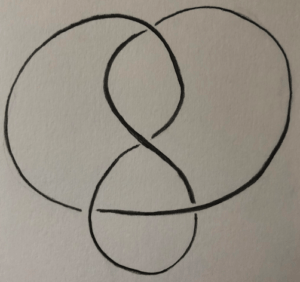Introduction

Here I present a way to construct a triangulation of $S^3-K$ where $K$  is the figure 8 knot.There are several ideas involved and also several major implications, such as a hyperbolic structure and the computation of the fundamental group. I won’t develop here those implications, but be focused on the construction of the triangulation.

How does the manifold look like

It is reasonable to start this post with some explanations about how the manifold, $S^3-K$ , should be thought.

The sphere is a compact manifold, and the knot is homeomorphic to a circle. So the difference is an open manifold of dimension three. It is an open manifold but it is really unconfortable in topology to work with open manifolds. So we must think of a way to simplify this situation.

If we take a closer look to the manifold structure, as given by a simple embedding of the knot in $S^3$ , It is easy to notice that the radius of the open balls muse decrease as we come closer to the knot. But, really, there is two way to understand this decreasing: the first way is the natural one if we keep the natural metric, but the second way is more interesting. We can set a metric such as the radius stay quite constant. But when we do that, we must push the knot to infinity, since the apparent radius come closer to zero. (Remember the infinity in $S^3$ is just a point.)

This metric is complete for the manifold: a convergent sequence can’t come close to the knot so there are no difficulty to have completeness.
Also, our triangulation won’t be quite a classical one. The vertices will be send to infinity, in such a way that they will represent the knot.

The strategy

To construct a triangulation of the figure 8 knot, there are quite several classical ways (notably some from Thurston) to proceed. But some are less general than others. I want to present here a way that can be fully generalized to other knots and even links. It is based on an article of William W. Menasco : Polyhedra Representation of Link Complements (1983).

The strategy will be the following.
We think the knot as standing on a plane. When we are far enough of the crossings, we simply have a line on the plane, and we will keep this that way. But when we are close to a crossing, the knot isn’t really standing on the plane: it’s going a bit above and below the plane, this is where we will have to work the most.
The idea to construct the triangulation is to think of two balloons, each one on a side of the plane. (In fact we see $S^3$ as separated in two components by a 2-sphere, thought as the plane.) Each balloon is progressively blown.
The balloons will give two open 3-cells, the plane will give the open 2-cells, and the knot will give (in a way described below) the open 1-cells and the 0-cells. (Remember that the knot will eventually be a 0-cell, and in fact the 0-cell.)

What happens close to a crossing

So the critical point is to study the blowing of a balloon close to a crossing.
A crossing is given by two segments, one above the other. The idea is to set an edge (so, a 1-cell and two 0-cells) between the two segments.
When we blow the ballon, it will reach the segment closer to It first. Then It will progressively be touching the edge two times and then reach the segment below. So if we think the edge as been dyed with a wet dye, the balloon will get two marks from the edge.Putting pieces together

With this procedure, we can construct the desired triangulation.

1. We encapsulate each crossing with a square with vertices touching the knot.
2. The edges between squares are left as there are.
3. When we have a crossing, we mark the edges of the square as if we were blowing a balloon. The edges are oriented as wished, but coherent in a way that we can shift an edge to the next one keeping the vertices on the knot.
4. When two squares are linked by a band, we can in fact identify the edges bordering the band and match the orientations such as there are coherent. The edges of the preceding two squares now become the same.
5. The bi-gons are collapsed since there are just contractible bands.After this steps, we should get a figure with 2-cells indicated by the edges, 1-cells given by the oriented edges of the squares, and 0-cells given by the knot.
To obtain the final step, we just have to think the edges of the knot which are not from the squares as shrunk. The two tetrahedra are each one given by :

• The 3-cell is the balloon chosen.
• The 2-cells are the areas delimited by at least 3 oriented edges (at all time). Do not forget the exterior face!
• The 1-cells and 0-cell are given by the squares remembering which edges to consider since only 2 out of 4 are from the balloon considered.